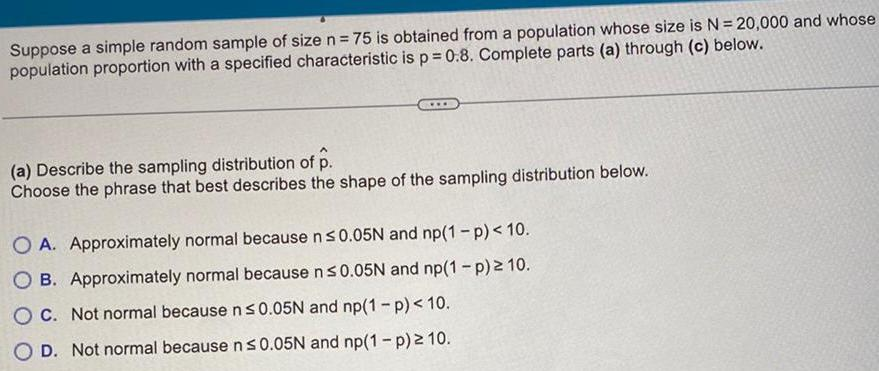Question:

# Suppose a simple random sample of size n 75 is obtained from

Last updated: 11/21/2023Suppose a simple random sample of size n 75 is obtained from a population whose size is N 20 000 and whose population proportion with a specified characteristic is p 0 8 Complete parts a through c below a Describe the sampling distribution of p Choose the phrase that best describes the shape of the sampling distribution below O A Approximately normal because n 0 05N and np 1 p 10 OB Approximately normal because n 0 05N and np 1 p 210 OC Not normal because n 0 05N and np 1 p 10 O D Not normal because n 0 05N and np 1 p 10